Construction - Perpendicular to a Line

# Construction - Perpendicular to a Line

GCSE(F), GCSE(H),

Geometric construction uses a pencil, ruler and a pair of compasses. To construct a line to be perpendicular (at right angles to) to another line:

from a point near the left hand end of the line (A), draw an arc above the line using a pair of compasses. Without changing the setting of the compasses, and from the same point, draw an arc below the line;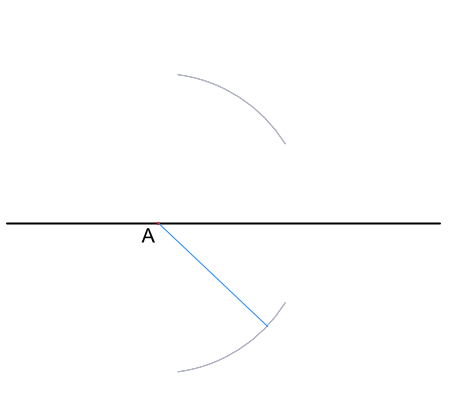from a point (B) toward the right hand end of the line, draw similar arcs above and below;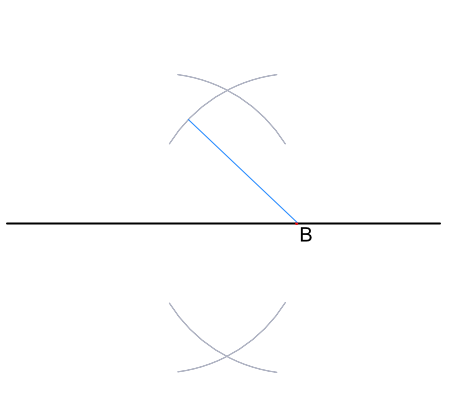join the two intersection points C-D for a perpendicular line.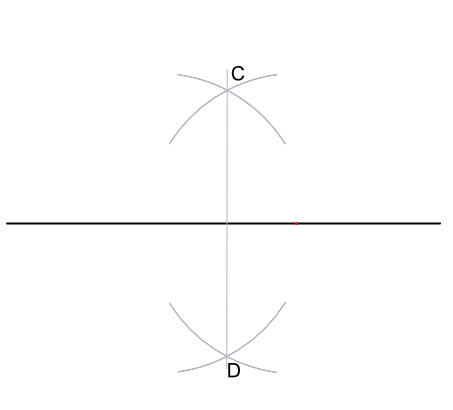The perpendicular will bisect (divide equally into two) the line between the points used to draw the arc (i.e. the perpendicular will be halfway along AB). This is known as a Perpendicular Bisector.

## Examples

1. Draw a vertical line at least 8 cm long. Draw a horizontal line that is perpendicular to the drawn line.

Answer: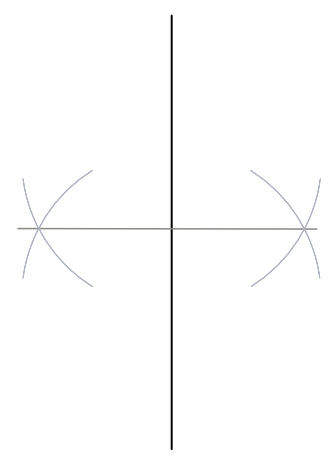Follow the same process as above, but turned 90º as the base line is now horizontal.

2. Explain what may have gone wrong with the construction of the perpendicular line, below: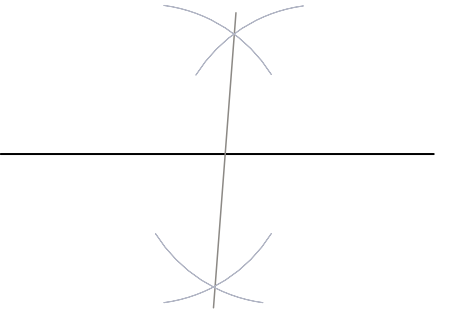Answer: Either the radius set 30 the compasses has changed when drawing the arc; or the centre point has changed when drawing an upper/lower pair of arcs.